•Hi Yanbo,

There can be multiple reasons for the behavior you see. It seems like you are solving a convex QCP. On this problem class, Barrier or interior point methods in general are known to perform very well. Regarding the MIPFocus setting, it might be that MIPFocus 3 performs additional model reformulations which due to shaky numerics can lead to numerical issues. Could you share the first and second iteration problems to make this issue reproducible? You can find more information on sharing files in the Community Forum in Posting to the Community Forum.

Best regards,
Jaromił

•Hi Jaromił,

First of all, thank you for your reply! The following is the first optimized. In fact, the second optimization only takes the result of the first optimization as input. This is the link to the LP file https://www.filemail.com/d/cytdqybsubmosoq

LP information with QC of

qc = [[[-178, -178, 100], [-178, 178, 100], [178, -178, 100], [178, 178, 100]]]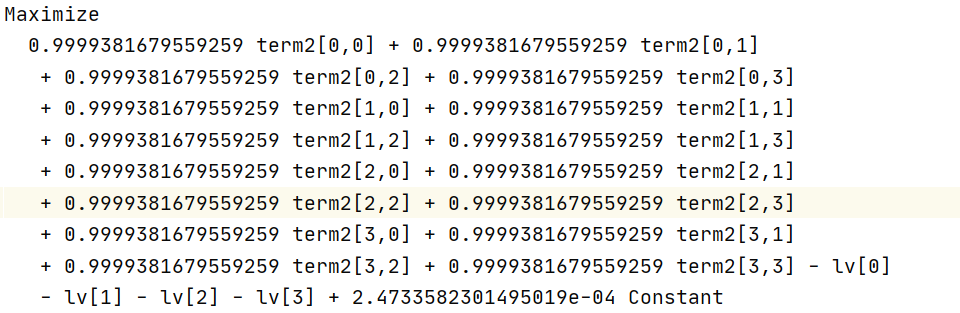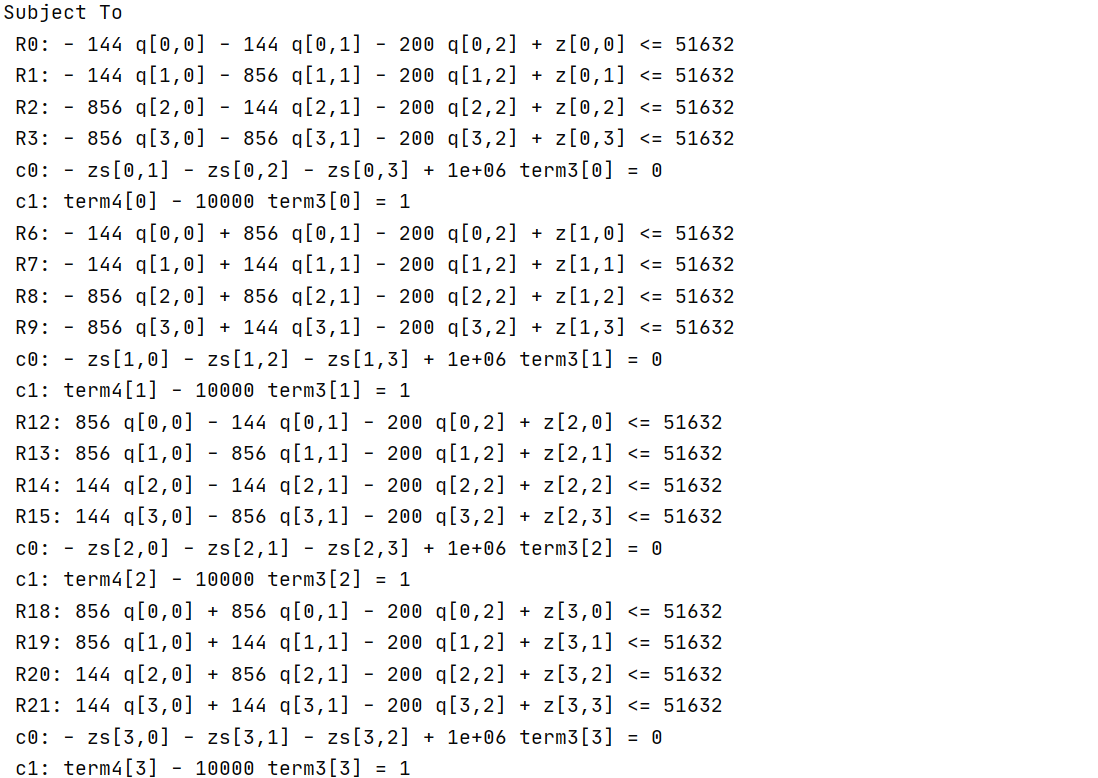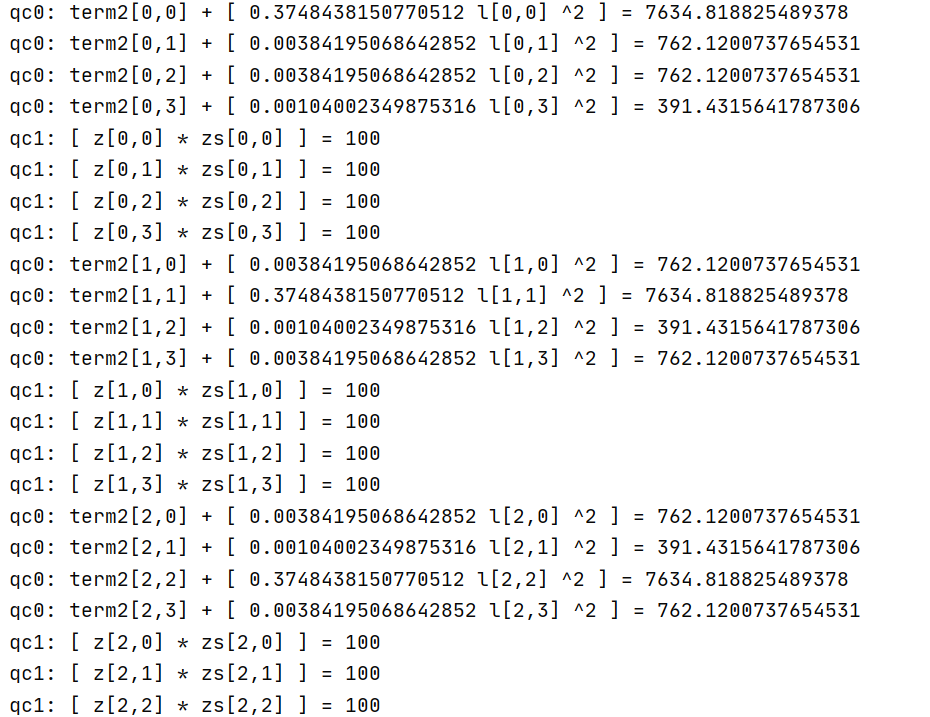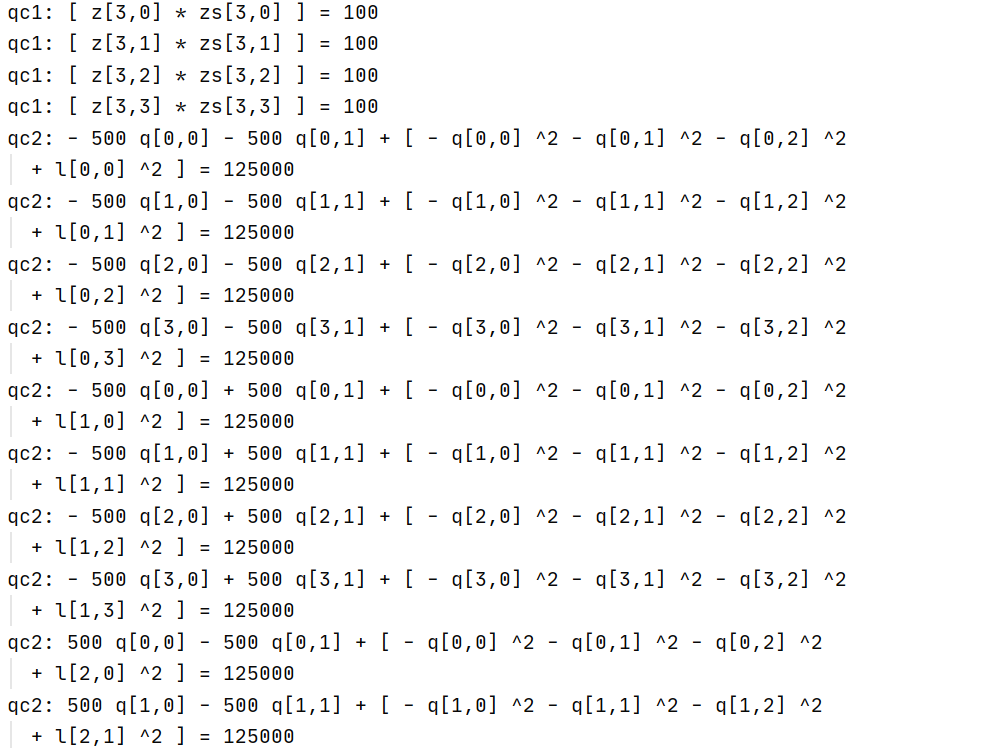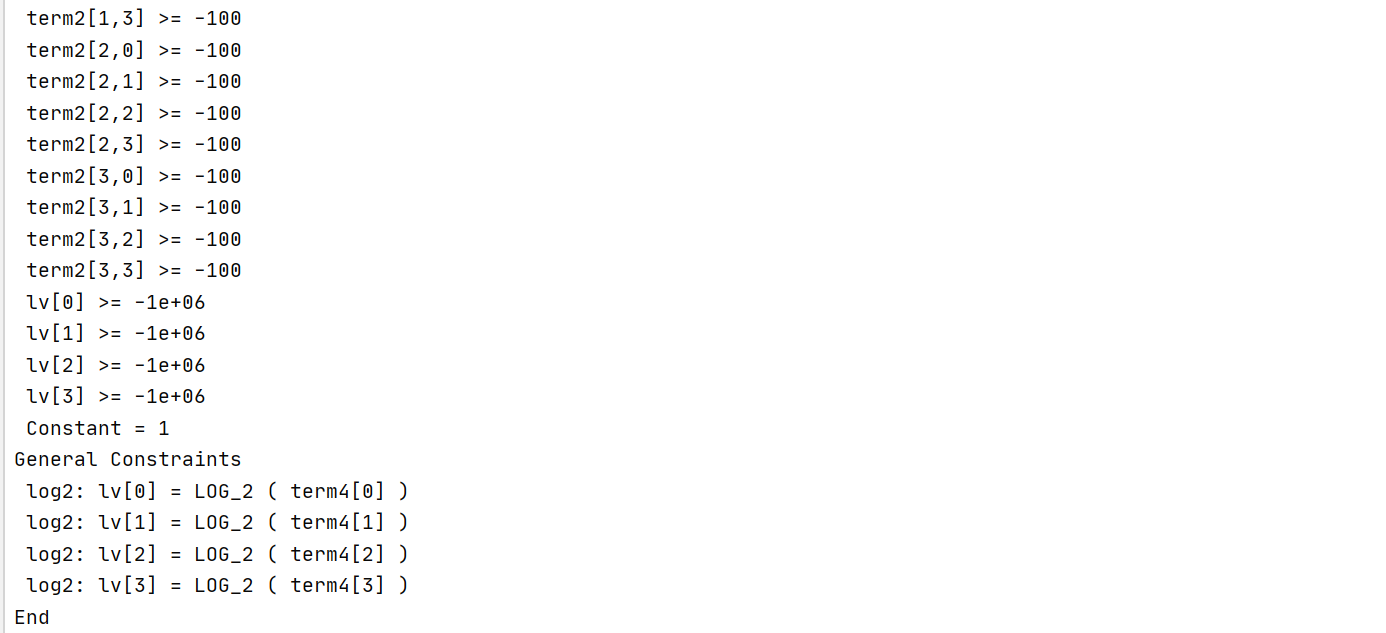It can be seen from the LP file that the decision variable Q in the model is an array containing four objects.

q = model.addVars(4, 3, lb=-300, vtype=GRB.CONTINUOUS, name='q')
• Combined with LP information and slack value of constraint C0, can it be considered that the max violation exceed tolerance problem is caused by C0？
• Is it max violation exceed tolerance  problem that leads to the failure of the second optimization?
model.addConstr(term3[k] * 1000000 == grb.quicksum(zs[k, j] for j in range(K) if j != k), "c0")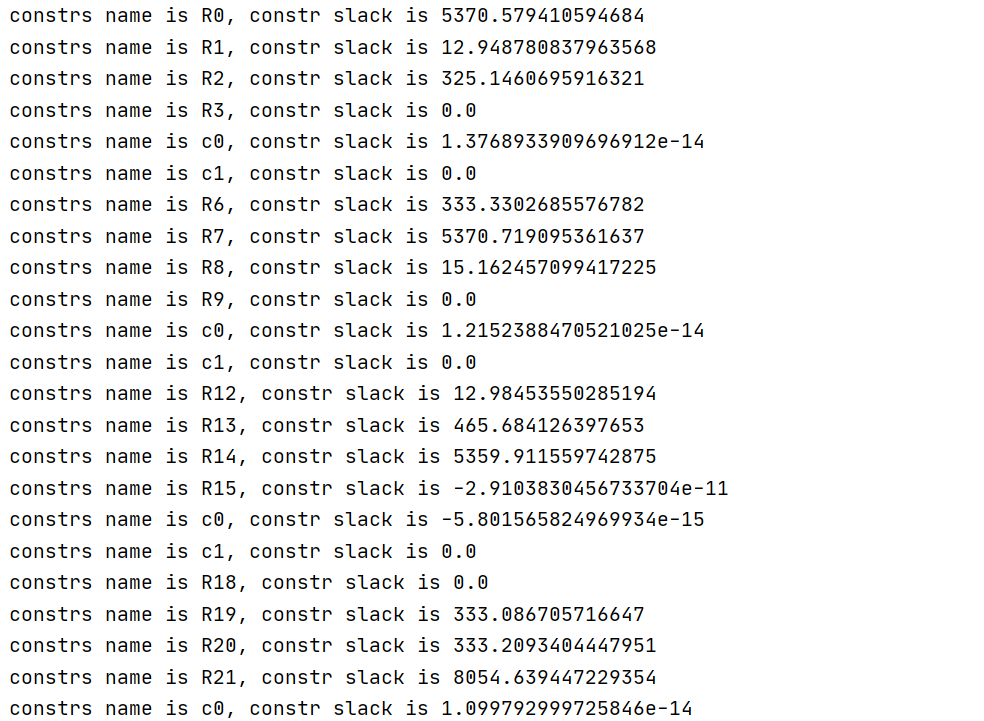•Hi Yanbo,

Yes, the issue you see is connected to the max violation exceed tolerance Warning.

You are using 4 general constraints in your model.

log2: lv = LOG_2 ( term4 )log2: lv = LOG_2 ( term4 )log2: lv = LOG_2 ( term4 )log2: lv = LOG_2 ( term4 )

However, you do not define any finite bounds for the $$\texttt{term4}$$ variables. This may lead to a weak piecewise-linear approximation of the general constraints and result in large constraint violations. It is recommended to always provide tight finite lower and upper bounds for variables participating in general constraints. In your case defining

term4 >= 0.1term4 >= 0.1term4 >= 0.1term4 >= 0.1 term4 <= 2term4 <= 2term4 <= 2term4 <= 2

seems to be enough to achieve normal convergence.

Best regards,
Jaromił

•Hi Jaromił ,

After adopting your suggestion, I set the upper and lower bounds for term4. What makes me happy is that after solver obtains the first optimization result, the second optimization can proceed smoothly and the gap value decreases. But it still can't get the results smoothly.

This is the time and result of the first run. From the information, gurobi quickly calculated the result.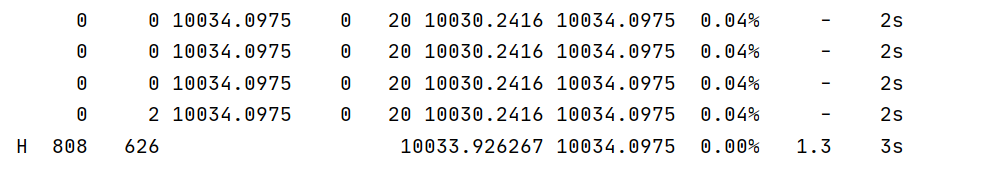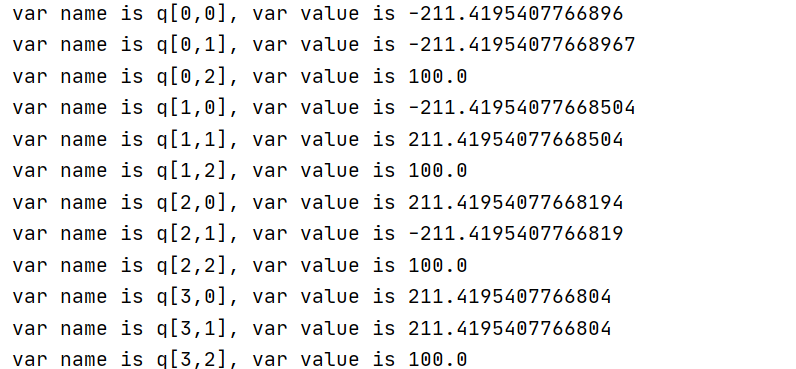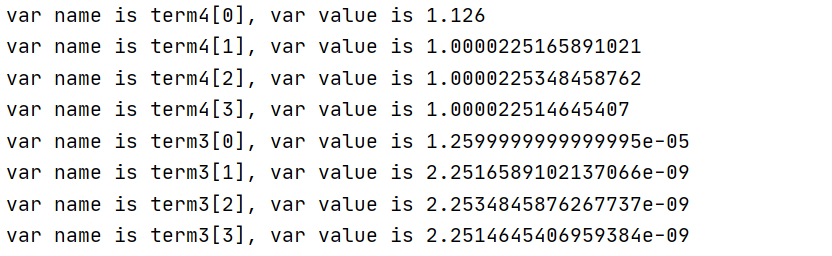The following is the second optimization process. Although the gap value and gap decrease compared with the previous case which I did not set the bounds for term4, the gap always stagnates at a value and it is difficult to continue to decrease.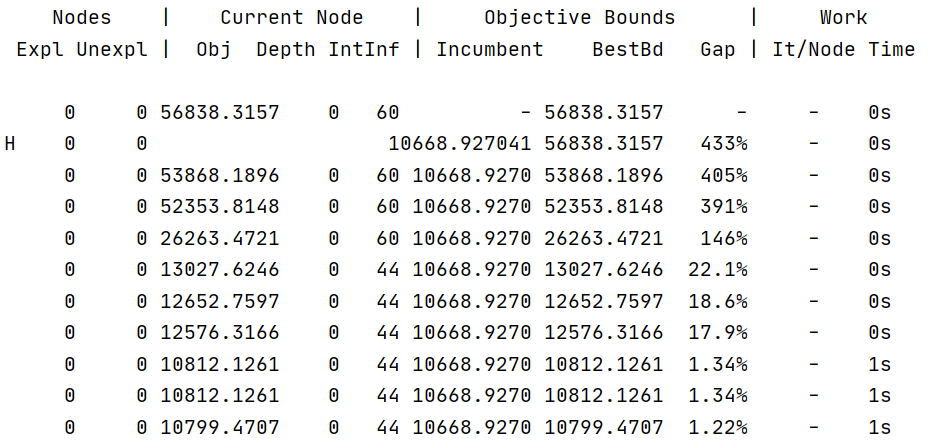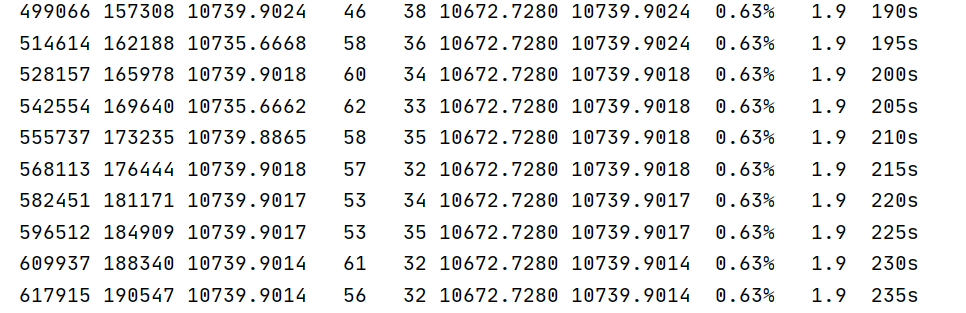Although I can get the second result by setting MIPGap, such as setting MIPGap to 0.006, the third optimization faces the same problem.

At the same time, I also found that there is a max violation exceed tolerance Warning in the first optimization.

• Is this warning caused by a problem with my constraints?

This is the first warning during optimization.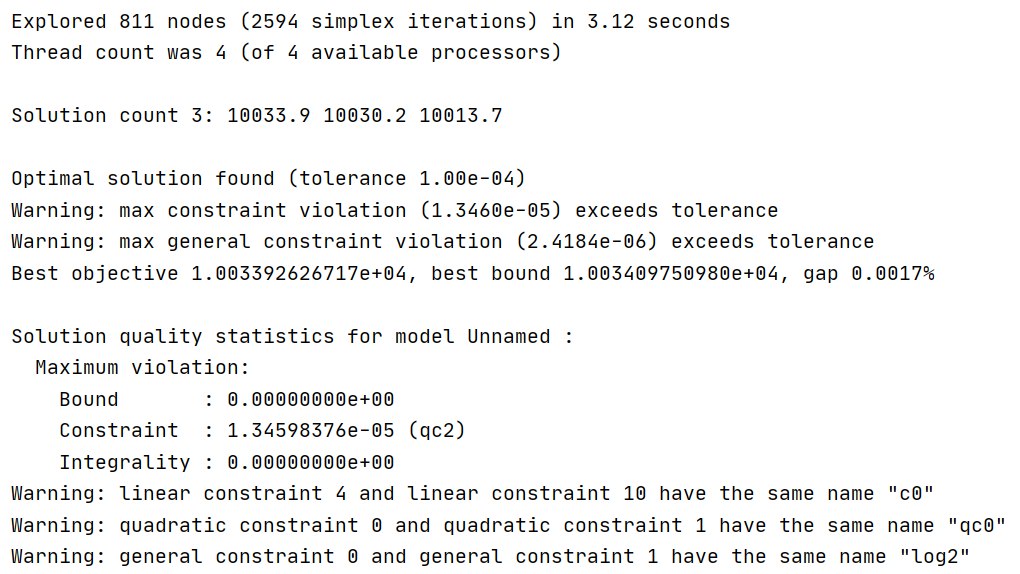Best regards,

Yanbo

•Hi Yanbo,

Could you provide the second optimization file as well? The Warning indicates that the issue is very likely caused by numerical issues with general constraints

Warning: max general constraint violation (2.4184e-06) exceeds tolerance

This then very likely propagates to other constraints.

At the same time, I also found that there is a max violation exceed tolerance Warning in the first optimization.

Is this warning caused by a problem with my constraints?

It is very likely caused by large difference in order of magnitude of the coefficient and right hand sides in the quadratic constraints. You could try to scale the coefficients. Our Guidelines for Numerical Issues provide good ideas of how to approach scaling. Alternatively, you could try setting the NumericFocus parameter to improve computational accuracy.

Best regards,
Jaromił

•Hi Jaromił,

Could you provide the second optimization file as well?

This is the LP model after I set MIPGap to 0.006. The reason why I set MIPGap is that in the second optimization process, it is difficult to reduce gap after reaching 0.006.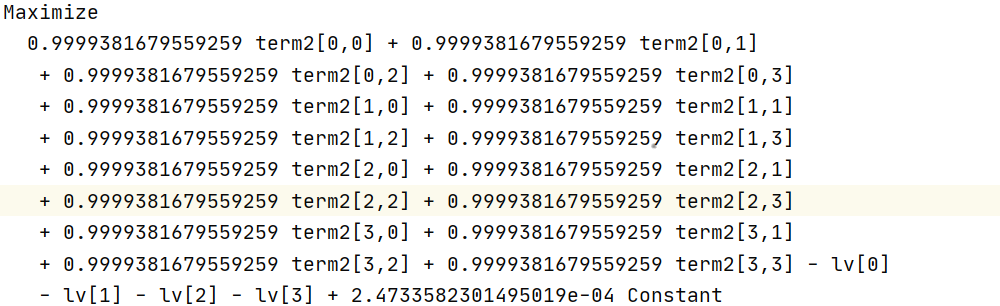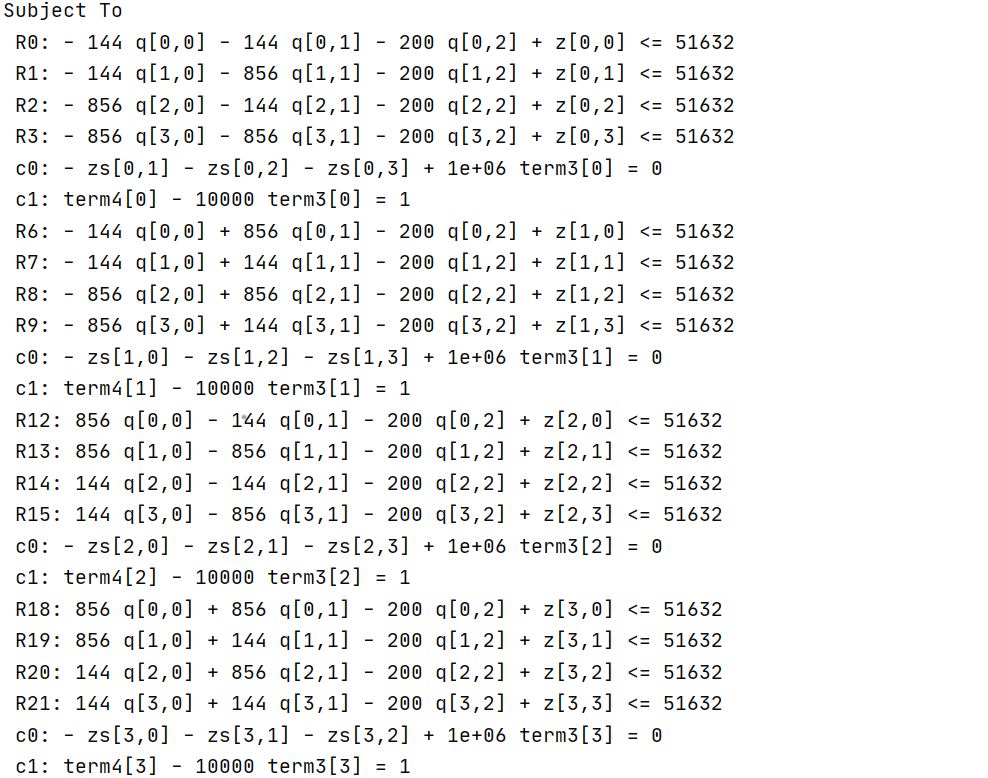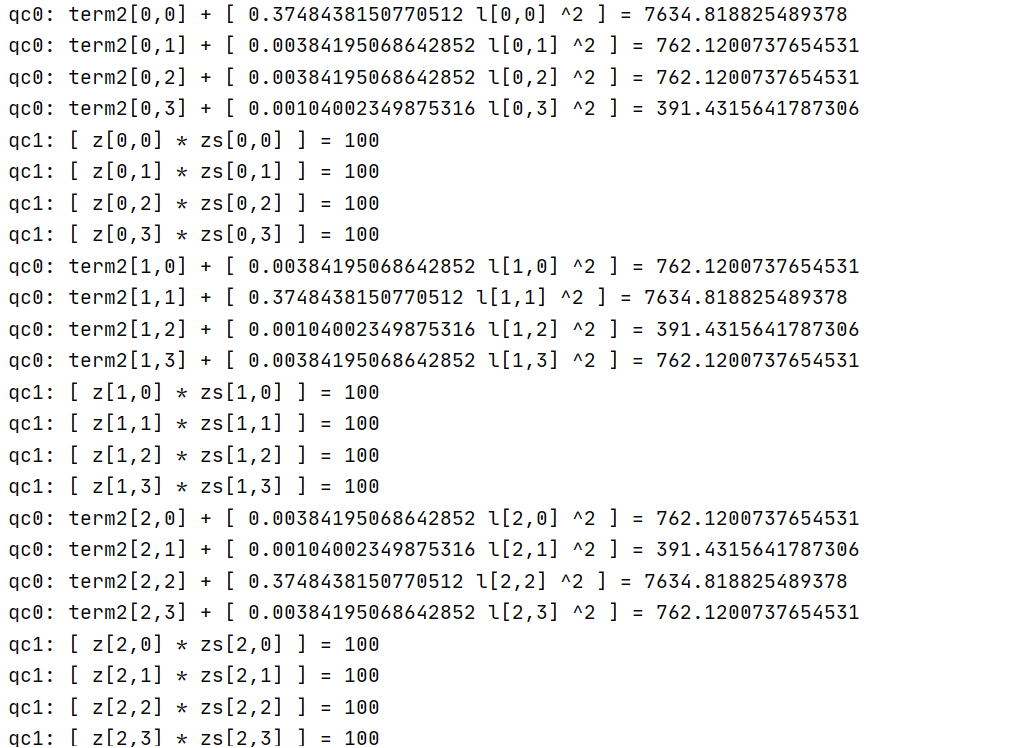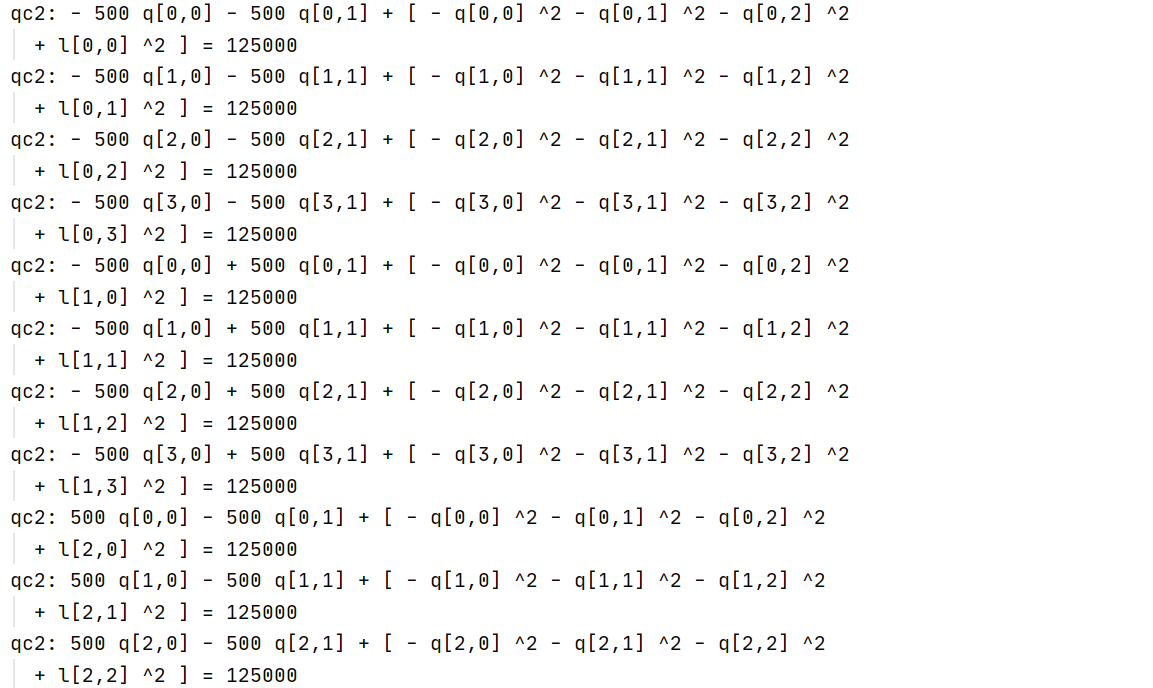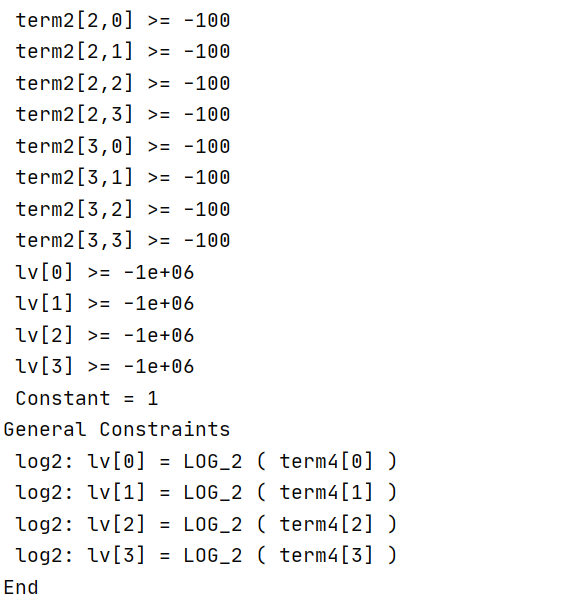There are some differences between the second optimized LP model and the first optimized LP model in the coefficient line of variables. From the information point of view, the coefficients of the second optimized LP model are more complex.

The above results are all the results of setting NumericFocus to 2.

• Could you know which constraints has numerical issues from the LP model and slack?
•Hi Yanbo,

In the latest screenshot of the model file, you are not setting any bounds for the $$\texttt{term4}$$ variables. However, this seems necessary to make your problem solve without constraint violations.

The above results are all the results of setting NumericFocus to 2.

Please note that the NumericFocus parameter has an effect on the optimization process and not on the model file itself. Thus, you should see different behavior in the log file when setting NumericFocus=2.

Could you know which constraints has numerical issues from the LP model and slack?

From the solution quality output, you can see that constraint named "qc2" is violated by 1.3e-05. Unfortunately, you have multiple constraints with the same name. You could provide unique names for all constraints and then you would see exactly which constraint is violated.

Altogether, the behavior here is most likely caused by a combination of not setting bounds for the $$\texttt{term4}$$ variables, the complexity of the problem, and its rather large differences in orders of magnitudes of the coefficients in quadratic constraints. You could try decreasing the FeasibilityTol parameter to improve computational accuracy even further.

Best regards,
Jaromił

•Hi Jaromił Najman,

Sorry, the LP information I provided above may be incorrect. The following is the correct LP file, and I set the boundary of term4.https://www.filemail.com/d/xefvvdrvyudqqtv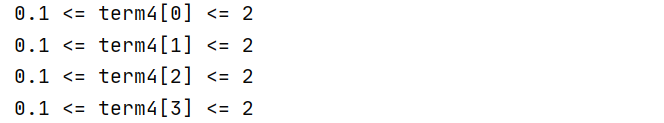Best regards,

Yanbo

•I put the slack output of the quadratic constraint.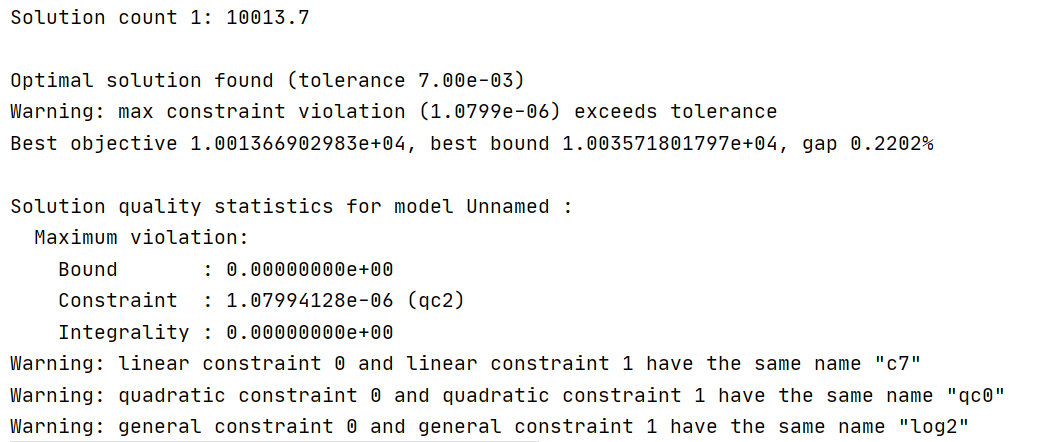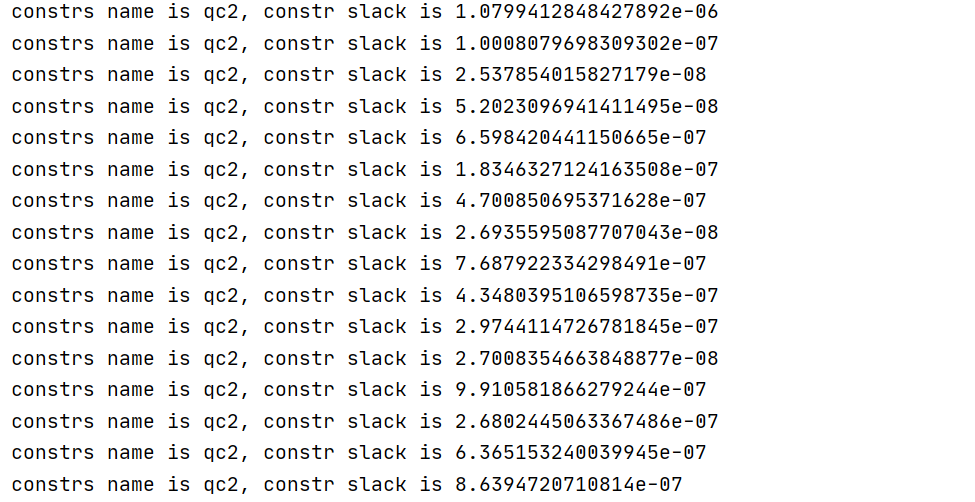• Is it the problem caused by constraint QC2? How can I modify QC2?
model.addConstr(l[k, j] * l[k, j] == rhs + s[k] ** 2 + s[k] ** 2 + s[k] ** 2, "qc2")

•Hi Yanbo,

Given that the problem has nonconvex terms together with general constraint and the FeasibilityTol is 1e-6, the violation you see is rather negligible and should be acceptable for most practical appliations. You could try decreasing the FeasibilityTol parameter or experiment with the NumericFocus parameter to improve the solution quality.

You could try providing tight lower and upper bounds for all variables participating in nonlinear terms, i.e., variables $$\texttt{l, q, z, zs}$$. You could also try to decrease the values of the RHS of the quadratic constraints. Would it be possible to use 125 instead of 125000 by going from, e.g., grams to kg? This would result in a different scale for the solution point but you can then scale the values back to your original order of magnitude of interest.

Best regards,
Jaromił

•Hi Jaromił,

Thanks to your guidance, I am getting closer and closer to success. The previous information shows that there may be a problem with the constraint QC2, so I try to decrease the values of the RHS of the QC2.

model.addConstr(l[k, j] * l[k, j] == rhs + s[k] ** 2 + s[k] ** 2 + s[k] ** 2, "qc2"
model.addConstr(l[k, j] * l[k, j] == (rhs + s[k] ** 2 + s[k] ** 2 + s[k] ** 2) * 0.00001, "qc2")

I just changed QC2. I want to see the effect first. Happily, each iteration went smoothly, and each optimization process did not have the previous warning about constraint violation except for the first optimization.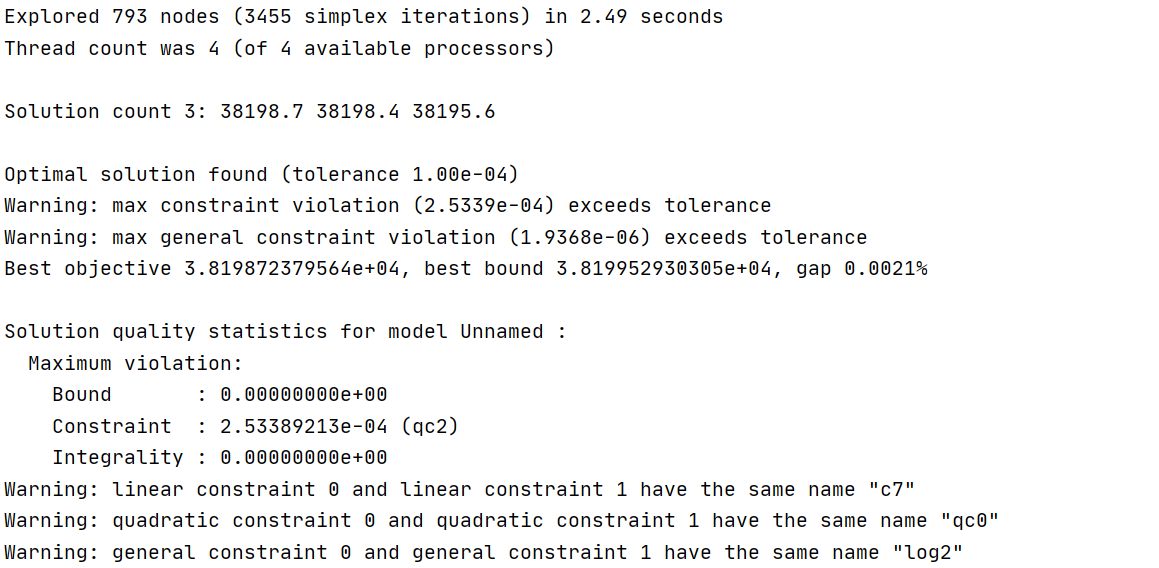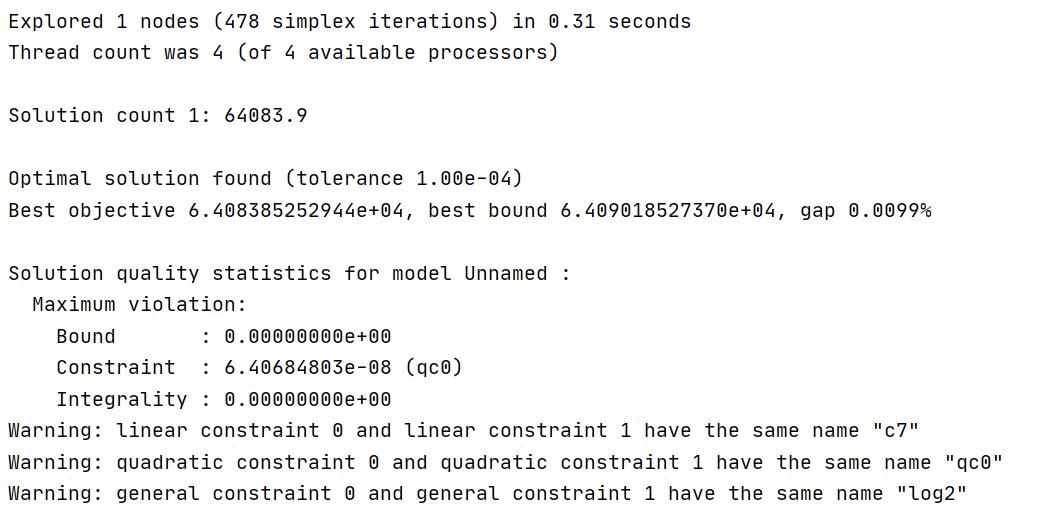However, only changing the right side of QC2 will lead to the change of L [K, J] in subsequent calculation. Specifically related to QC0.Then I multiply L [K, J] in QC0 by 100000 to ensure the correctness of the whole model.

model.addConstr(term2[k, j] == -1 * 1.44 * 10000 * (l[k, j] * l[k, j] - np.linalg.norm(qc[j] - s[k]) ** 2) / dc[k, j] ** 4, "qc0")
model.addConstr(term2[k, j] == -1 * 1.44 * 10000 * (l[k, j] * l[k, j] * 100000 - np.linalg.norm(qc[j] - s[k]) ** 2) / dc[k, j] ** 4, "qc0")

When the same problem occurs, There was no warning in the first optimization, but there was a warning in the second iteration, but there were also results. But the third optimization can not get the results smoothly.

first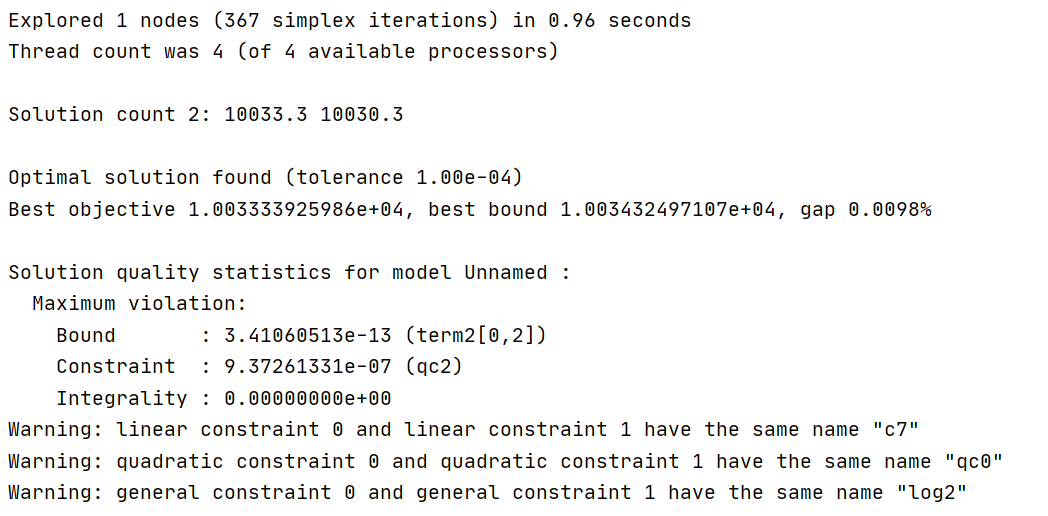second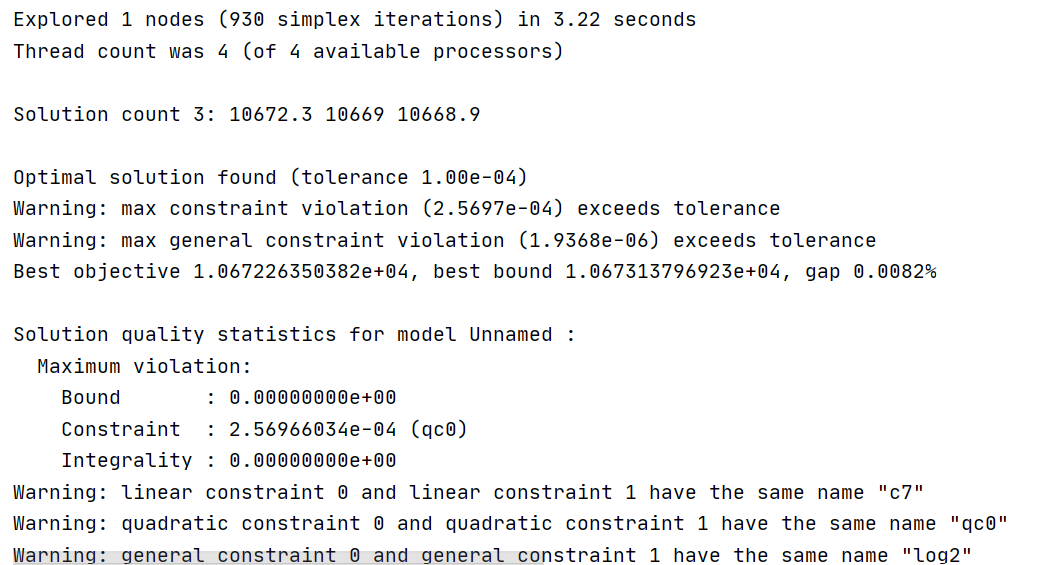third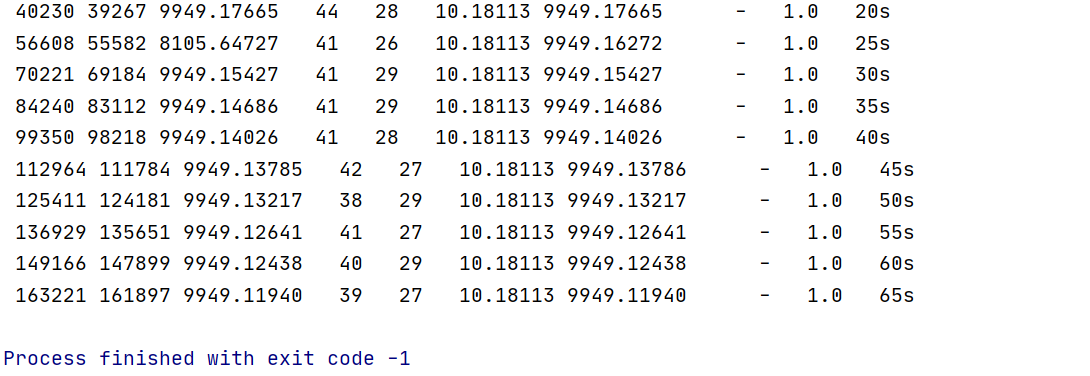• Is my handling of QC2 and QC0 wrong?
•Hi Yanbo,

I don't think that multiplying the problematic constraint with 1e-6 or 1e+6 is the correct way to go as you only shift the tolerance in one or the other direction without really addressing the core issue.

It would be best if you would be able to formulate the constraint as

model.addConstr(term2[k, j] == -1 * 1.44 * (l[k, j] * l[k, j] - np.linalg.norm(qc[j] - s[k]) ** 2) / dc[k, j] ** 4, "qc0")

and work with the new order of magnitude in values of $$\texttt{term2}$$. Alternatively, you could adjust the order of magnitude for values of variables $$\texttt{l}$$. However, it is recommended to keep the bounds of nonlinear variables as tight as possible and in best case within 4 orders of magnitude.

What exactly changes in the third optimization problem compared to the previous two? Are the numerics getting worse? Could you post the coefficient statistics for the third optimization problem?

Best regards,
Jaromił

•Hi Jaromił,

With reference to the ideas you gave me, I changed the coefficients of various constraints again. Fortunately, the iterative algorithm can proceed smoothly, but the results do not seem to be convergent. But before that, let me introduce my whole restriction change so that you can better understand my problem.

First, the dc is calculated according to the given qc, which is the result of the previous optimization.

dc[k, j] = np.linalg.norm(qc[j] - s[k])model.addConstr(term2[k, j] == -1 * 1.44 * (l[k, j] * l[k, j] - np.linalg.norm(qc[j] - s[k]) ** 2) / dc[k, j] ** 4, "qc0")sum += term2[k, j] / term1[k]

Probably because QC0 involves the 4th power of dc, gap value will not decrease for a long time when solver is solved.

Then, I reduce dc [K, J] to one tenth and multiply 100 on the molecule of RHS of QC0, In order to keep the model unchanged, I modified the coefficients of term2 expression.

dc[k, j] = np.linalg.norm(qc[j] - s[k]) / 10model.addConstr(term2[k, j] == -1 * 1.44 * 100 * (l[k, j] * l[k, j] - np.linalg.norm(qc[j] - s[k]) ** 2) / dc[k, j] ** 4, "qc0")sum += term2[k, j] / (term1[k] * 1000000)

In this way, the iterative algorithm can run smoothly.  Similar operations are as follows.

model.addConstr(z[k, j] * zs[k, j] == 1, "qc1")model.addConstr(term3[k] == grb.quicksum(zs[k, j] for j in range(K) if j != k), "c0")model.addConstr(term4[k] == term3[k] + 1, "c1")model.addGenConstrLogA(term4[k], lv[k], 2, "log2")sum += -1 * lv[k]
model.addConstr(z[k, j] * zs[k, j] == 100, "qc1")model.addConstr(term3[k] * 100 == grb.quicksum(zs[k, j] for j in range(K) if j != k), "c0")model.addConstr(term4[k] == term3[k] + 1, "c1")model.addGenConstrLogA(term4[k], lv[k], 2, "log2")sum += -1 * lv[k]

The following is the LP model and information of the last iteration algorithm. https://www.filemail.com/d/ytgtgwvwvnljpnl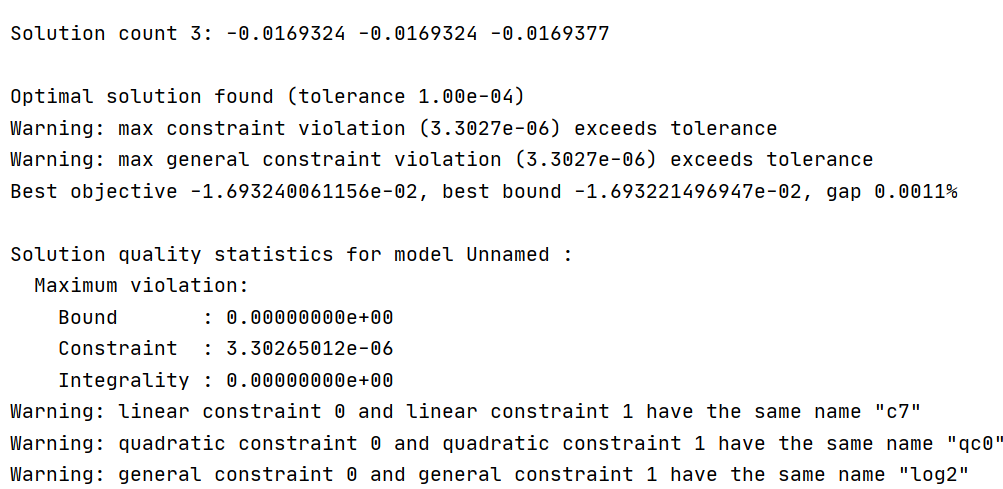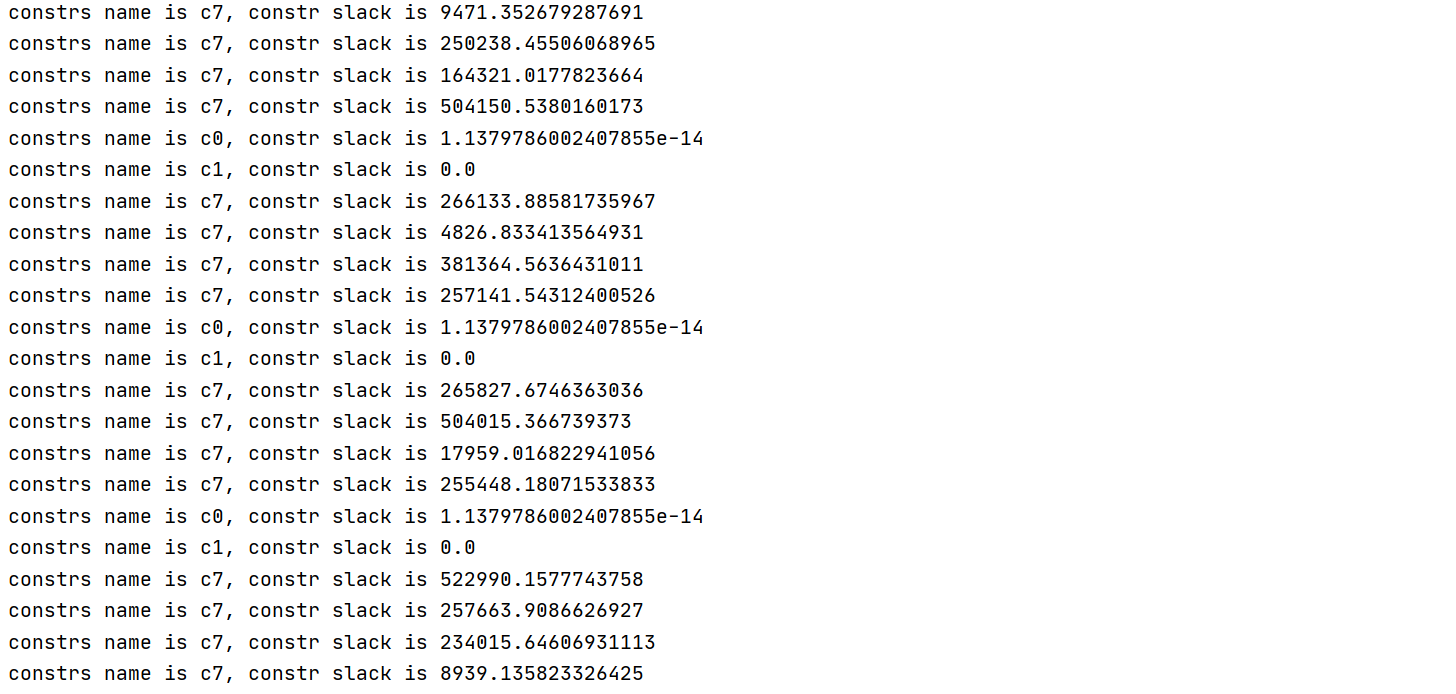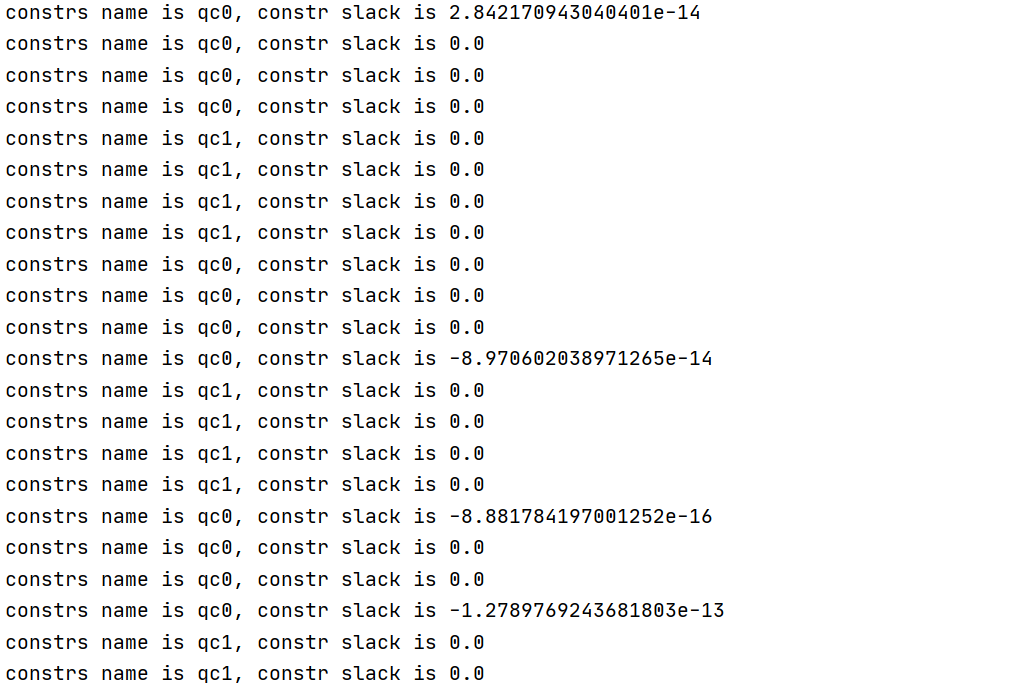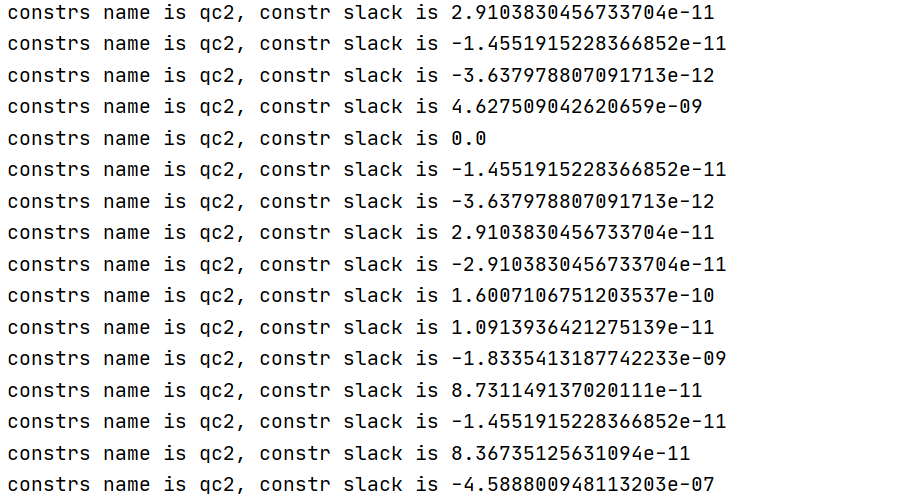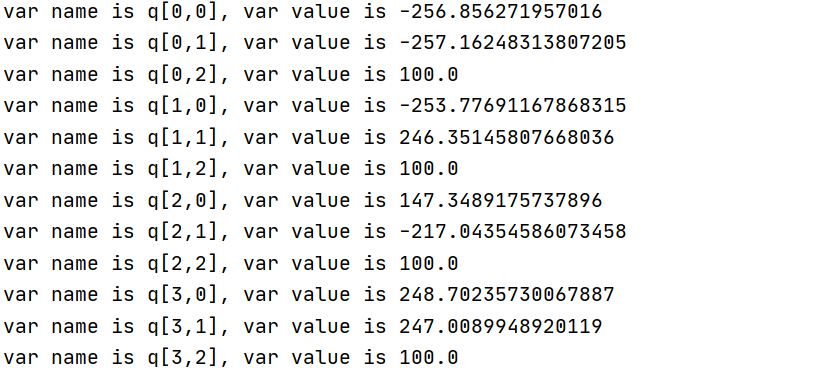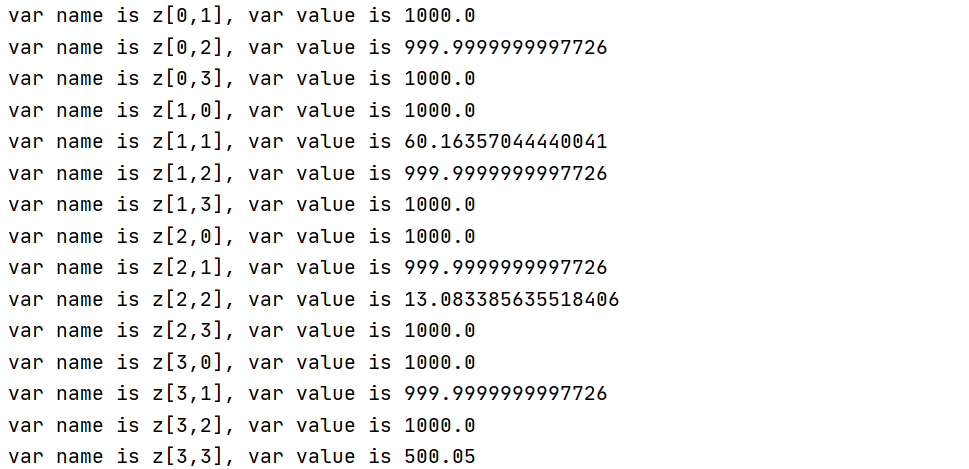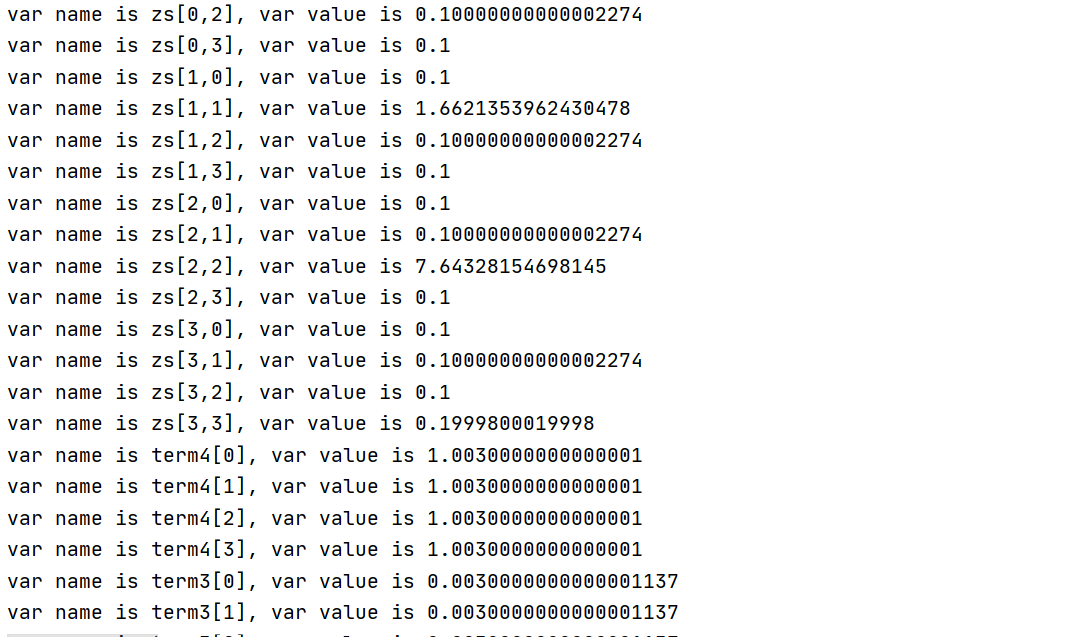• Is it max violation problem that causes the non convergence of the iterative algorithm?
• Will it improve if I try to change the unit of decision variable q and input qc of each iteration, like 200 meters to 2 ten meters?
•Hi Yanbo,

I just tested the latest LP file you provided with Gurobi v9.1.2 on a Linux machine and the algorithm converges smoothly.

[...]* 2301   981              12      -0.0168447   -0.01684  0.00%   2.2    0sCutting planes:  RLT: 6Explored 2301 nodes (5202 simplex iterations) in 0.49 secondsThread count was 8 (of 8 available processors)Solution count 1: -0.0168447 No other solutions better than -0.0168447Optimal solution found (tolerance 1.00e-04)Best objective -1.684470261578e-02, best bound -1.684470261578e-02, gap 0.0000%

Are you using the latest version of Gurobi? Which OS are you using?

Is it max violation problem that causes the non convergence of the iterative algorithm?

Usually, such small violations as in your case are not a problem for iterative algorithms. They may become problem if you use the solution point of a previous run for the next iteration run. Due to the constraint violations, this point may not be accepted as feasible initial point. In this case you could try the following:

• Increase the number of FuncPieces used for the piecewise-linear approximation of the $$\log_2$$ function or decrease the FuncPieceError to improve the accuracy. I would recommend working with FuncPieces first.
• Increase the FeasibilityTol to 1e-5. It is usually not recommended to increase feasibility tolerances. In particular the smallest meaningful coefficient should be at least one order of magnitude above the feasibility tolerance, i.e., for FeasibilityTol=1e-5, the smallest coefficient should be 1e-4. However, in this specific case, the problem at hand is very nonlinear. Thus accounting for the nonlinear by slightly increasing feasibility tolerances may be reasonable and can in most cases be properly argued from a practical point of view.

Will it improve if I try to change the unit of decision variable q and input qc of each iteration, like 200 meters to 2 ten meters?

Yes, this very likely will improve the numerical behavior. After the problem has been solved, you can scale the value of variable $$\texttt{q}$$ back to its original scaling, i.e., in this case multiply $$\texttt{q}$$ with 100 after the optimization has been completed.

I hope this helps and we can get closer to a solution of the numerical issues.

Best regards,
Jaromił

•Hi Jaromił,

Maybe I didn't express it clearly. I mean that the last iteration process can converge to get the final result of the current iteration, but the whole iterative algorithm is not convergent. My goal is to maximize a function and ensure that if the function is concave, the result of each optimization should be bigger than the previous optimization.

However, in my external iterative algorithm, there will be violations of the above mentioned conditions，like this.

1.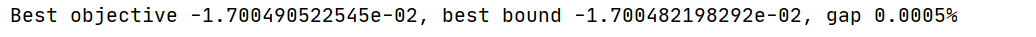2.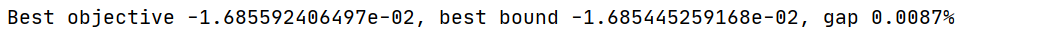3.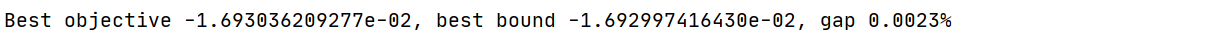Best objective is not monotonic increase.

• Is max violation problem in the iteration process causing this problem?

Warning messages appear in many optimization processes of the iterative algorithm.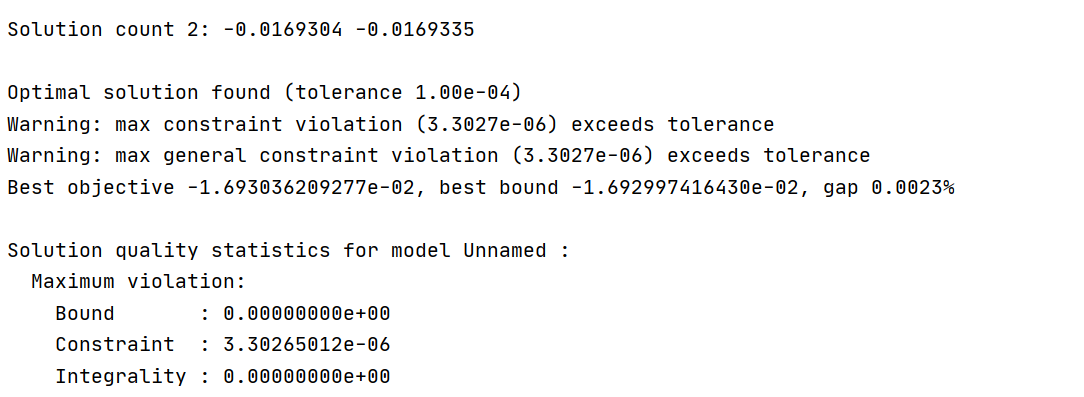•Hi Jaromił,

After several days of debugging, I have made great progress with your help. The following is a supplement to my previous answer.

The first is the version of gurobi I use.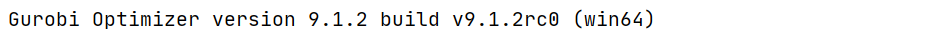And then, I set the FuncPieces to 15000. The iterative algorithm can run smoothly this time. The optimal target value of each optimization has better stability than before.

However, there are still numerical fluctuations between each optimization. In other words, in the whole iterative algorithm, the first few iterations can ensure that the optimal solution of the objective function is increasing. But with the progress of the iteration, the best target value of the next iteration is not strictly increasing compared with the best target value of the previous iteration (I'm solving a maximization function).

When I look at the solution information, I find that there are often warnings about constraints QC2 in each optimization process.

for k in range(K):    for j in range(K):        rhs = QuadExpr()        for i in range(3):            rhs += q[j, i] * q[j, i] - 2 * s[k][i] * q[j, i]        model.addConstr(l[k, j] * l[k, j] == rhs + s[k] ** 2 + s[k] ** 2 + s[k] ** 2, "qc2")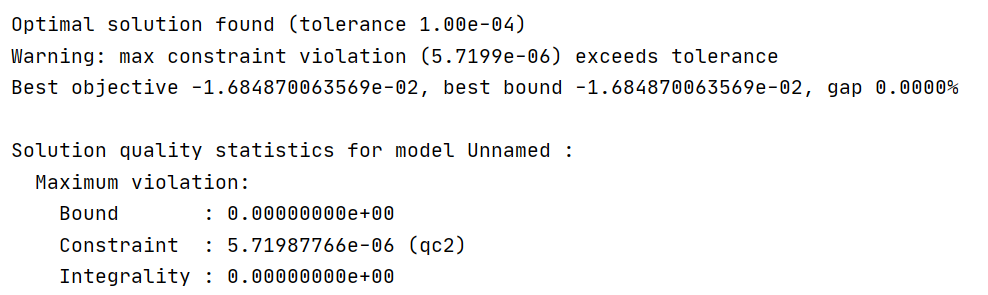• How to solve the tolerance violation problem of equality constraints?

I tried to convert the unit of q from meters to 10 meters, trying to solve this problem.

s = np.array([[-250, -250, 0], [-250, 250, 0], [250, -250, 0], [250, 250, 0]])q = model.addVars(4, 3, lb=-300, ub = 300, vtype=GRB.CONTINUOUS, name='q')
s = np.array([[-25, -25, 0], [-25, 25, 0], [25, -25, 0], [25, 25, 0]])q = model.addVars(4, 3, lb= -30, ub = 30, vtype=GRB.CONTINUOUS, name='q')

However, a warning of tolerance violation is displayed constraints QC2 in the solution information.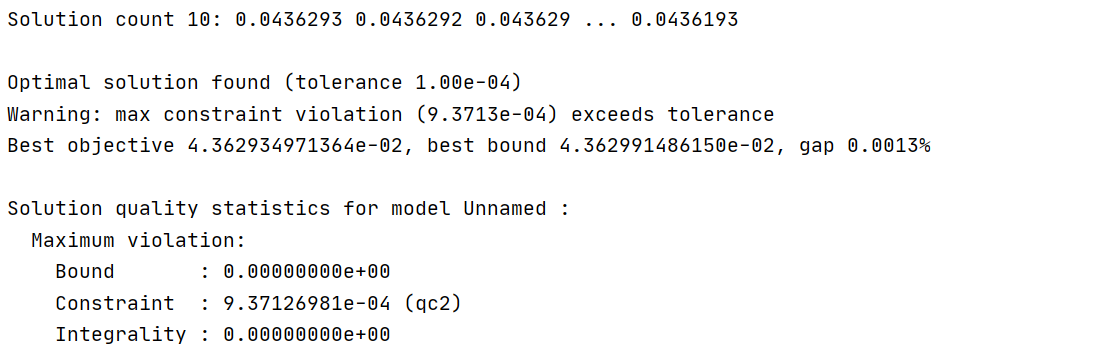•Hi Yanbo,

Good to hear that you are making progress.

I suppose that the numerical problems are connected to the small coefficients you are using in your model. We recommend to set the FeasibilityTol at least one order of magnitude lower than the smallest meaningful coefficient in your model. In my previous message, I recommended to increase the FeasibilityTol despite the small coefficients to hopefully avoid problematic numerics. It seems like this did not fully help. This time, could you try reducing it to, e.g., 1e-8? It is possible that you will still get constraint violation but these should be way smaller.

In best case, you could try to re-scale your coefficient to be > 1e-4. Did you try experimenting with the NumericFocus parameter?

I also noticed that, e.g.,

term2[0,1] + [ 3.84195e-05 l[0,1] ^2 ] = 7.443977977527512

and the objective coefficient of $$\texttt{term2}$$ is of value ~1e-6. It might help to scale the objective coefficients to be of order of magnitude around ~1e-1 to actually catch the real impact of variables $$\texttt{term2}$$.

Best regards,
Jaromił

•Hi Jaromił,

I set FeasibilityTol  to 1-e8. As you think, the tolerance violation warning still exists, but the quality of the solution has been improved. But there is also the problem that the optimal value of the objective function is not strictly increasing.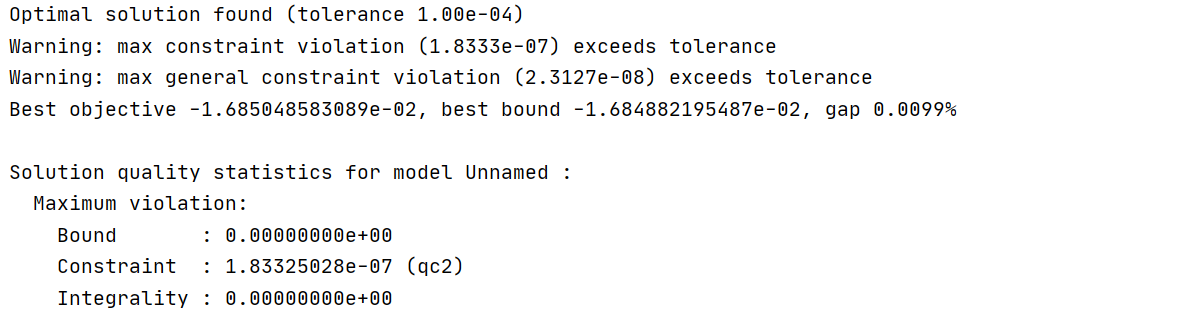Did you try experimenting with the NumericFocus parameter?

All my previous results are based on the parameter NumericFocus of 2. If I want to get a more accurate solution process, when I set NumericFocus to 3, solver will be very difficult to get results. About all the parameters I use.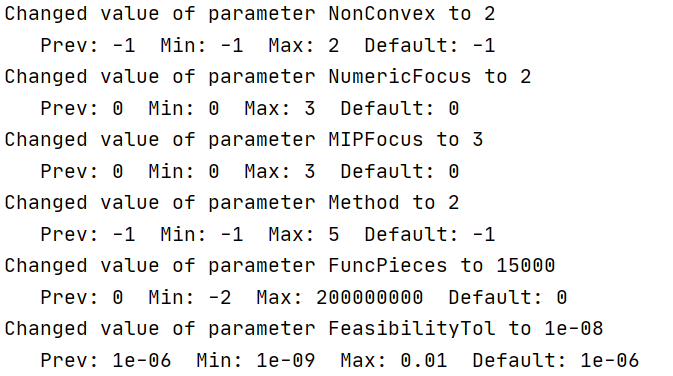When I scale decision variables, I find it difficult for me to scale variables in the form of my current objective function. My objective function looks like this.

Max linear function ( - q^2 ) - log ( 1 / q^2)

When I reduce the coefficient of q, the existence of logarithmic function makes it difficult for me to reduce the coefficient. In other words, linear function( 0.1 * - q^2) - log(  1 / 0.1 * q^2), It has a different mathematical meaning from the original function. The coefficients between the two sub functions are not scaled equally.

• How can the scaling  coefficient be effective when the function has a log function?

Facing this problem, I transformed the objective function to get a new approximate function. Two new sub functions can get the same solution as before the transformation, the linear function and log function. In more detail, I convert the log function to the liner function.

Similarly, I replaced the formula of the LHS in the original objective function with the formula of the RHS in the figure below, so as to build an approximate function.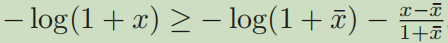I thought that by avoiding the log function, numerical and performance problems could be improved. However, when the two sub functions are calculated together, the calculation time is still unacceptably long, but gap does decline all the time.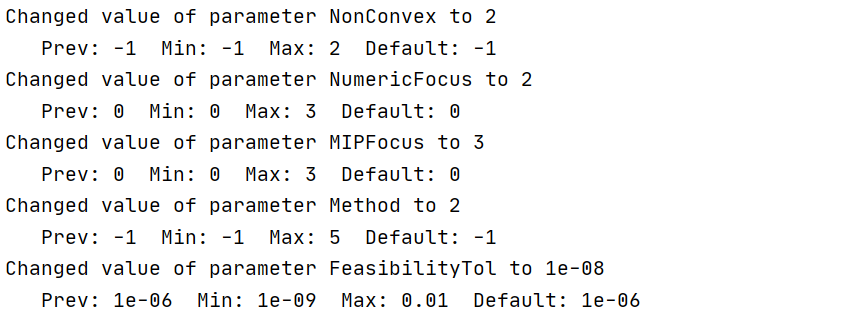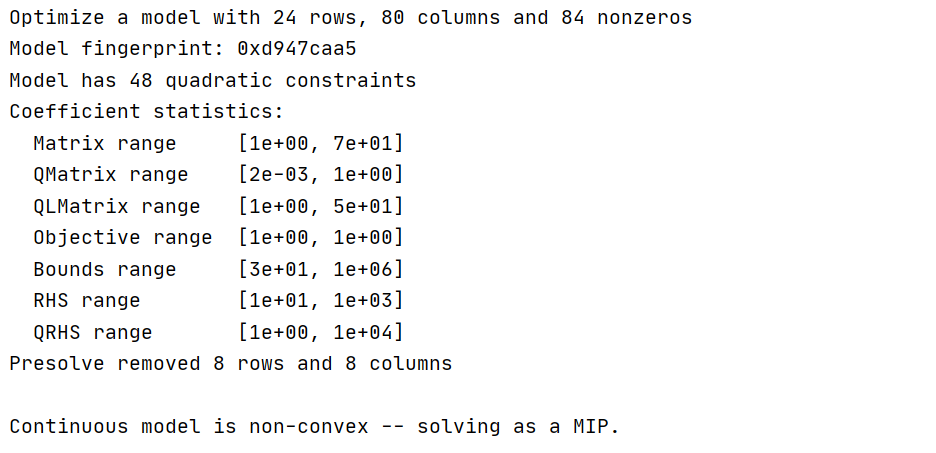PS. After I change the initial value, I find that it is difficult for gurobi to get the result when the initial value is quite different from the optimal solution. However, if the initial solution is close to the optimal solution, gurobi can get the result in a limited time.

Max constraint violation tolerancec still exists. And this is lp file.https://www.filemail.com/d/dvvpldjhldzlbog

• Does the converted model improve the numerical or computational performance compared with the previous model?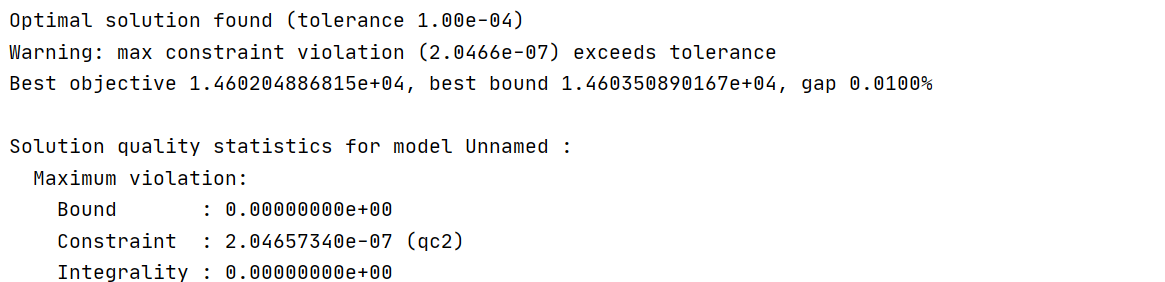•Hi Yanbo,

Let's take a step back and review what you have so far.

The file model3.lp you provided in your last message solves quickly (~10s) with Gurobi version 9.1.2 on my personal Mac even with FeasibilityTol=1e-9 and MIPGap=0.01%. There are no constraint violations if I set FeasibilityTol to either 1e-7, 1e-8, or 1e-9. There are small violations for the default value 1e-6.

The numerics of model3.lp look good so I don't think that further scaling is needed.

How can the scaling coefficient be effective when the function has a log function?

Coefficient scaling does not affect your particular objective function. However, the bounds of the input variables $$q$$ have an impact on the piecewise-linear approximation of the $$\log$$ function.

Let's try to focus on the fact that the objective function does not increase from one iteration to the other.
What is the theoretical guarantee that should make the objective function increase? Could you provide 2 models where the objective function should increase from model1 to model2 but it does not?

Best regards,
Jaromił

•Hi, Jaromił

After several days of debugging, although I can get a result through the model, I have different results when I split the model and calculate separately, which leads me to doubt the correctness of the solution and some problems in the intermediate calculation.

What is the theoretical guarantee that should make the objective function increase? Could you provide 2 models where the objective function should increase from model1 to model2 but it does not?

Let me describe my algorithm in detail and briefly. This is a simple example. If I want to solve a model like Max x ^ 2 (3 < x < 8). We know that this is not a convex optimization problem because the objective function is a maximized convex function. But x^2 is convex about x. Then, at any point a, the first-order Taylor expansion x ^ 2 has x ^ 2 > = 2 * a * x - a ^ 2， because x ^ 2 is a convex function. So I construct an approximate function of x ^ 2 at any point a in the feasible region, x ^ 2 > = 2 * a * x - a ^ 2.

Then, Max x ^ 2 (3 < x < 8)-------------------> Max 2 * a * x - a ^ 2 (3 < x < 8), a is any point in the feasible solution domain.

The new problem is a strictly convex optimization problem. Therefore, after a given point a, the global optimal solution can be found. The construction of the whole algorithm is as follows.

1. Set r = 0, and iteration tolerance  t > 0.

2. Initialize a( 0 ) for a in feasible region,(3 < a < 8)

3.  repeat

4. update a(r  + 1 ) == x * by solving Max 2 * a(r) * x - a(r)  ^ 2 (3 < x < 8)
5. Set :r =r + 1
6. until default tolerance reached
7. Output a(r)

It can be verified that the optimal objective value of problem is non-decreasing with the iteration number r and it is upper-bounded. Therefore, the sequence of objective values of problem converge as r goes to infinity.

Returning to my question, I find that the following two pieces of code will produce different results.

def gurobi_demo2(qc):    model = grb.Model('')    s = np.array([[-150, -150, 0], [-150, 150, 0], [150, -150, 0], [150, 150, 0]])    q = model.addVars(4, 3, lb=-300, ub=300, vtype=GRB.CONTINUOUS, name='q')    z = model.addVars(4, 4, vtype=GRB.CONTINUOUS, name='z')    zs = model.addVars(4, 4, vtype=GRB.CONTINUOUS, name='zs')    term3 = model.addVars(4, vtype=GRB.CONTINUOUS, name='term3')    term2 = model.addVars(4, 4, lb=-100000, vtype=GRB.CONTINUOUS, name='term2')    l = model.addVars(4, 4, vtype=GRB.CONTINUOUS, name='l')    dc = np.array([[0, 0, 0, 0], [0, 0, 0, 0], [0, 0, 0, 0], [0, 0, 0, 0]])    for k in range(4):        for j in range(4):            dc[k, j] = np.linalg.norm(qc[j] - s[k])    term1 = {}    for k in range(K):        term1[k] = 1        for o in range(K):            term1[k] += 1 / (np.linalg.norm(qc[o] - s[k]) ** 2)    model.update()    sum = LinExpr()    for k in range(K):        sum += math.log(term1[k]) * 10000        for j in range(K):            model.addConstr(term2[k, j] == -1 * 10000 * (l[k, j] * l[k, j] - np.linalg.norm(qc[j] - s[k]) ** 2) / dc[k, j] ** 4, "qc0")            sum += term2[k, j] / term1[k]    model.setObjective(sum, sense=GRB.MAXIMIZE)    # -------------------------------------------------------------------    for k in range(K):        model.addConstr(q[k, 2] == 100, "c2")    # np.linalg.norm(q[j] - s[k])    for k in range(K):        for j in range(K):            rhs = QuadExpr()            for i in range(3):                rhs += q[j, i] * q[j, i] - 2 * s[k][i] * q[j, i]            model.addConstr(l[k, j] * l[k, j] == rhs + s[k] ** 2 + s[k] ** 2 + s[k] ** 2, "qc2")    model.setParam('NonConvex', 2)    model.optimize()    for v in model.getVars():        print('var name is {}, var value is {}'.format(v.VarName, v.X))    return [[q[i, j].X for j in range(3)] for i in range(4)]
def main():    qc = [[[-200, -200, 100], [-200, 200, 100], [200, -200, 100], [200, 200, 100]]]    while True:        qc.append(gurobi_demo2(qc[-1]))        print(qc)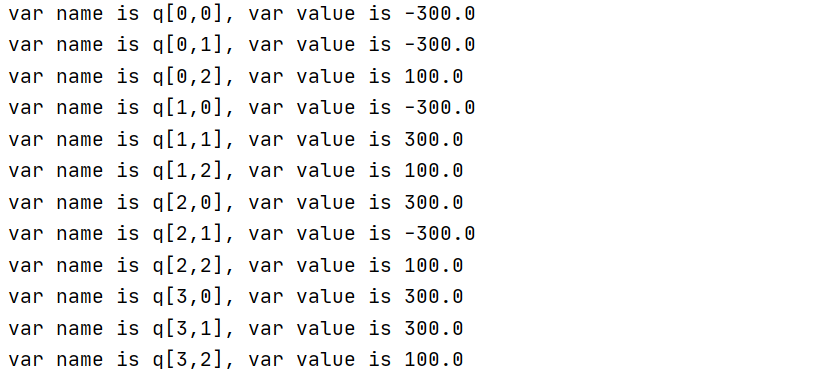def gurobi_demo2(qc):    model = grb.Model('')    s = np.array([[-150, -150, 0], [-150, 150, 0], [150, -150, 0], [150, 150, 0]])    q = model.addVars(4, 3, lb=-300, ub=300, vtype=GRB.CONTINUOUS, name='q')    z = model.addVars(4, 4, vtype=GRB.CONTINUOUS, name='z')    zs = model.addVars(4, 4, vtype=GRB.CONTINUOUS, name='zs')    term3 = model.addVars(4, vtype=GRB.CONTINUOUS, name='term3')    term2 = model.addVars(4, 4, lb=-100000, vtype=GRB.CONTINUOUS, name='term2')    l = model.addVars(4, 4, vtype=GRB.CONTINUOUS, name='l')    dc = np.array([[0, 0, 0, 0], [0, 0, 0, 0], [0, 0, 0, 0], [0, 0, 0, 0]])    for k in range(4):        for j in range(4):            dc[k, j] = np.linalg.norm(qc[j] - s[k])    term1 = {}    for k in range(K):        term1[k] = 1        for o in range(K):            term1[k] += 1 / (np.linalg.norm(qc[o] - s[k]) ** 2)    model.update()    sum = LinExpr()    for k in range(K):        sum += math.log(term1[k]) * 10000        for j in range(K):            model.addConstr(term2[k, j] == -1 * (l[k, j] * l[k, j] - np.linalg.norm(qc[j] - s[k]) ** 2) / dc[k, j] ** 4, "qc0")            sum += term2[k, j] / term1[k]    model.setObjective(sum, sense=GRB.MAXIMIZE)    # -------------------------------------------------------------------    for k in range(K):        model.addConstr(q[k, 2] == 100, "c2")    # np.linalg.norm(q[j] - s[k])    for k in range(K):        for j in range(K):            rhs = QuadExpr()            for i in range(3):                rhs += q[j, i] * q[j, i] - 2 * s[k][i] * q[j, i]            model.addConstr(l[k, j] * l[k, j] == rhs + s[k] ** 2 + s[k] ** 2 + s[k] ** 2, "qc2")    model.setParam('NonConvex', 2)    model.optimize()    for v in model.getVars():        print('var name is {}, var value is {}'.format(v.VarName, v.X))    return [[q[i, j].X for j in range(3)] for i in range(4)]
def main():    qc = [[[-200, -200, 100], [-200, 200, 100], [200, -200, 100], [200, 200, 100]]]    while True:        qc.append(gurobi_demo2(qc[-1]))        print(qc)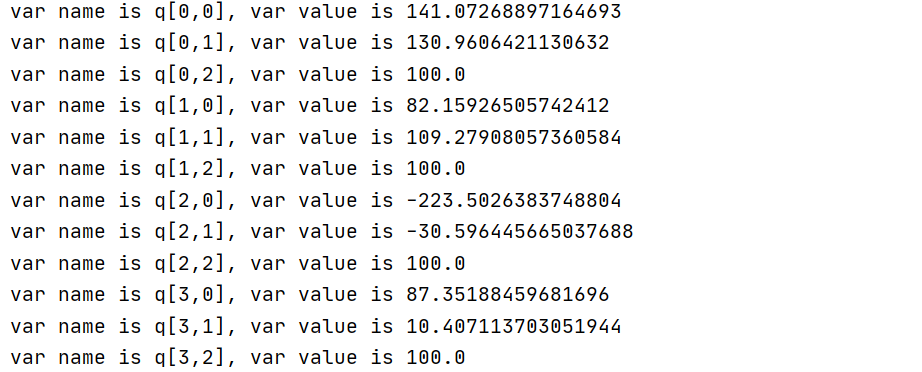• Why do two pieces of code have different results? Their difference is only the coefficient before the decision variable.
model.addConstr(term2[k, j] == -1 * 10000 * (l[k, j] * l[k, j] - np.linalg.norm(qc[j] - s[k]) ** 2) / dc[k, j] ** 4, "qc0")
model.addConstr(term2[k, j] == -1 * (l[k, j] * l[k, j] - np.linalg.norm(qc[j] - s[k]) ** 2) / dc[k, j] ** 4, "qc0")

When I used CVX to solve the second code, different results appeared. It bothers me that the same model produces different results. I don't know which result is right.

•This is the code of CVX.

import cvxpy as cpimport numpy as npimport mathK = 4s = np.array([[-150, -150, 0], [-150, 150, 0], [150, -150, 0], [150, 150, 0]])R_r = 0def cvx_demo(ipc):    ir = [None] * K    ir1 = [None] * K    term1 = [ * K] * K    t = [[None] * K] * K    for k in range(K):        ik = 0        for j in range(K):            ik += 1 / ((np.linalg.norm(ipc[j] - s[k])) ** 2)        ir1[k] = ik    q = []    for k in range(K):        q.append(cp.Variable(shape=(3)))    objfunc = []    for k in range(K):        for j in range(K):            term1[k][j] += -1 * (cp.norm(q[j] - s[k]) ** 2 - (cp.norm(ipc[j] - s[k]) ** 2)) / (np.linalg.norm(ipc[j] - s[k]) ** 4)            objfunc.append(term1[k][j] / (1 + ir1[k]))    objfunc.append(10000 * math.log(ir1[k] + 1))    constr = []    for k in range(K):        constr.append(q[k] == 100)        for j in range(3):            constr.append(q[k][j] >= -300)            constr.append(q[k][j] <= 300)    prob = cp.Problem(cp.Maximize(sum(objfunc)), constr)    prob.solve()    return [qv.value for qv in q]def main():    qc = [[[-200, -200, 100], [-200, 200, 100], [200, -200, 100], [200, 200, 100]]]    count_iter = 0    while True:        print(count_iter)        count_iter += 1        qc.append(cvx_demo(qc[-1]))        print(qc)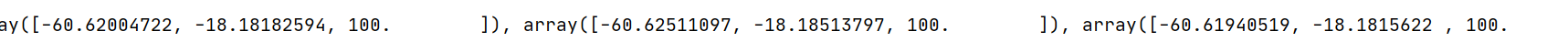•Moreover, I found that in the calculation process, there will always be warnings about the following restrictions. Is there a better way to express the relationship between decision variables q and s?

# np.linalg.norm(q[j] - s[k])    for k in range(K):        for j in range(K):            rhs = QuadExpr()            for i in range(3):                rhs += q[j, i] * q[j, i] - 2 * s[k][i] * q[j, i]            model.addConstr(l[k, j] * l[k, j] == rhs + s[k] ** 2 + s[k] ** 2 + s[k] ** 2, "qc2")
cp.norm(q[j] - s[k])
•Hi Yanbo,

Thank you for the detailed description. The algorithm sounds a bit like a specialized Newton's method for optimization.

I have a few questions which came up to my mind.

How do you make sure that your algorithm converges to the correct global maximum? For example if you want to solve

$\max x^2\\ x \in [-2,8]$
and set the initial point to $$-1$$, your approximation will provide you the solution point at $$x=-2$$. From this point, you cannot "jump" to the other side of the parabola $$x^2$$ to reach the global maximum at $$x=8$$. Or is it OK for your algorithm to find a local maximum?

I can imagine that the monotonicity criterion works for convex feasible sets. However, the models you shared have a nonconvex feasible set. Do you have a theoretical guarantee that the monotonicity property still holds even for nonconvex feasible sets? Or is the nonconvex feasible set actually convex but cannot be recognized as such by Gurobi?

Why do two pieces of code have different results? Their difference is only the coefficient before the decision variable.

When I used CVX to solve the second code, different results appeared. It bothers me that the same model produces different results. I don't know which result is right.

By multiplying the term by $$10000$$, you are changing optimal solution values of variables $$\texttt{term2}$$, which then affect the values of the $$\texttt{l}$$ variables, which ultimately affect the solution values of the $$\texttt{q}$$ variables. Additionally, you are shifting the feasibility tolerances of these equality constraints. Moreover, CVX performs a set of reformulations before passing the problem to Gurobi, which may as well affect the optimization process. Altogether I would use the second constraint formulation without the multiplication with $$10000$$ in this case.

Moreover, I found that in the calculation process, there will always be warnings about the following restrictions. Is there a better way to express the relationship between decision variables q and s?

As long as the violations are very small, e.g., 2e-6 for a FeasibilityTol of 1e-6, they can be acceptable for highly nonlinear models.

If possible, you could try to formulate the equality constraints as inequality constraints which would make them rotated second order cone constraints. This is only possible if the model formulation allows it, i.e., if the objective function pushes the variables in the correct direction. Inequality constraints are usually easier to solve in nonlinear optimization.

Best regards,
Jaromił

•Hi Jaromił

Due to some personal reasons, I'm sorry to reply to you for so few days llate.

How do you make sure that your algorithm converges to the correct global maximum?

In introducing this algorithm, I ignored the condition that x needs to be greater than 0. In fact, for the decision variables in this algorithm, X is greater than 0. Furthermore, this algorithm will convert a nonconvex function into a monotone approximation function, and most of the approximation functions are linear.

However, the models you shared have a nonconvex feasible set. Do you have a theoretical guarantee that the monotonicity property still holds even for nonconvex feasible sets?

Theoretically, the feasible set of this function needs to be convex. Could you explain in detail why you think there is a nonconvex feasible set?

If possible, you could try to formulate the equality constraints as inequality constraints which would make them rotated second order cone constraints. This is only possible if the model formulation allows it, i.e., if the objective function pushes the variables in the correct direction. Inequality constraints are usually easier to solve in nonlinear optimization.

Thank you very much for your advice. Formulating the equality constraints as inequality constraints in my case, sometimes it is very effective, but sometimes it doesn't work well.

Best regards,

Yanbo

•Hi Yanbo,

Theoretically, the feasible set of this function needs to be convex. Could you explain in detail why you think there is a nonconvex feasible set?

Nonlinear equality constraint, such as $$z_1 \cdot z_2 = 100$$ already define a nonconvex set. This is also the reason why I asked about turning the nonlinear equality constraints into inequalities. If you don't set the NonConvex parameter to 2 and Gurobi throws the error

Error 100021: ... Set NonConvex parameter to 2 to solve model.

then this means that Gurobi did not recognize the problem to be a convex one. Given, your linear objective function (or after reformulation maximizing a concave function), this means that the feasible set may be nonconvex. At least Gurobi does not recognize the feasible set to be convex. Given the equality constraints

qc2: - 50 q[0,0] - 50 q[0,1] + [ - q[0,0] ^2 - q[0,1] ^2 - q[0,2] ^2   + l[0,0] ^2 ] = 1250

I don't see how the feasible set can be convex. However, making the above an inequality constraint

qc2: [ - q[0,0] ^2 - q[0,1] ^2 - q[0,2] ^2 + l[0,0] ^2 ] >= 1250

and forcing all $$\texttt{q}$$ variables to be $$\geq 0$$, turns it into a rotated second order cone constraint which is convex.

Thank you very much for your advice. Formulating the equality constraints as inequality constraints in my case, sometimes it is very effective, but sometimes it doesn't work well.

Using nonlinear inequalities instead of nonlinear equalities whenever possible makes it easier for optimization solvers to recognize convexity so it definitely makes sense that you see positive effects.

Best regards,
Jaromił

•Hi  Jaromił,

With your help, I have solved most of the problems I have encountered before. Thank you very much.

Nonlinear equality constraint, such as z1⋅z2=100 already define a nonconvex set.

I didn't pay attention to this constraint before because I use this constraint to express a convex function， details are as follows.

When I minimize log(1 + 1/x) which is a convex  function respect to x, for x>0. Because GUROBI does not support the decision variable to appear in the denominator. In other words, log(1 +1 / x) is a convex function, but GUROBI can hardly recognize that it is a convex function. I introduced a new variable y and add a constraint which make x * y = 1. The next, I minimize log(1 + y), st. x * y =1, x>0. But I find log(1 + y) is a concave function respect y. In order to avoid this circumstances, how can I reformulate this problem(Min log(1 + 1 / x))? What's more, when I handled the function with 1 / x, is there any other way besides using constraint (y * x =1)?

qc2: - 50 q[0,0] - 50 q[0,1] + [ - q[0,0] ^2 - q[0,1] ^2 - q[0,2] ^2   + l[0,0] ^2 ] = 1250

I don't see how the feasible set can be convex. However, making the above an inequality constraint

qc2: [ - q[0,0] ^2 - q[0,1] ^2 - q[0,2] ^2 + l[0,0] ^2 ] >= 1250

and forcing all q variables to be 0, turns it into a rotated second order cone constraint which is convex.

For this reformulation, I also have some question. 1250 in qc2 is l? Where are the -50q[0,0] and- 50[0,1]?

Best regards,

Yanbo

•Hi Yanbo,

With your help, I have solved most of the problems I have encountered before. Thank you very much.

I am happy to hear that you are making progress.

In order to avoid this circumstances, how can I reformulate this problem(Min log(1 + 1 / x))? What's more, when I handled the function with 1 / x, is there any other way besides using constraint (y * x =1)?

I understand, thank you for the clarification. Unfortunately, it is currently not possible in Gurobi to reformulate the function $$f(x)=\log(1+\frac{1}{x})$$ in a way that the model remains convex. However, what you could try as an alternative is to use the addGenConstrPWL method to use a piecewise-linear approximation of $$f$$. To generate a piecewise-linear approximation, you first have to introduce finite tight bounds on $$x$$ and then compute point pairs $$(x,f(x))$$. You can have a look at the documentation on piecewise-linear functions and the piecewise.py example for more details. I think 1000 point pairs should be a good start. You might want to add more if necessary. This may improve the overall behavior of the model.

For this reformulation, I also have some question. 1250 in qc2 is l? Where are the -50q[0,0] and- 50[0,1]?

I dropped the linear part $$-50q_{0,0} - 50q_{0,1}$$ because it is not allowed in a second order cone constraint. If you cannot drop the linear part from the constraints, then please simply ignore this advice. Still, turning the equality constraint into an inequality constraint may lead to improved performance and optimization behavior.

Best regards,
Jaromił

•Hi Jaromił,

it is currently not possible in Gurobi to reformulate the function f(x)=log(1+1x)f(x)=log⁡(1+1x) in a way that the model remains convex. However, what you could try as an alternative is to use the addGenConstrPWL method to use a piecewise-linear approximation of f.

In the field of wireless communication, researchers will handle with a large number of functions like log (1 + 1 / x), In cvxpy, user can use the internal  function cp.inv_ pos to express this function, e.g. cp.log(1 + cp.inv_pos(x)). I hope gurobi can also add such functions, which will be more convenient to use. In addition, I also will learn  addGenConstrPWL method and piecewise-linear functions to using gurobi more skillfully.

At last, Thank you very much for your help. If I have any questions in the future, how can I contact you?

Best regards,

Yanbo

•Hi Yanbo,

I cannot promise that or when Gurobi will support additional nonlinear functions but we will certainly take it into consideration.

At last, Thank you very much for your help. If I have any questions in the future, how can I contact you?

I am glad that I could help. It is best you open a new Community Post in our Forum and I will certainly find it.

Best regards,
Jaromił